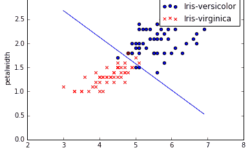## Implementing logistic regression learner with pythonLogistic regression is the next step from linear regression. The most real-life data have a non-linear relationship; thus, applying linear models might be ineffective. Logistic regression is capable of handling non-linear effects in prediction tasks. You can think of many different scenarios where logistic regression could be applied. There can be financial, demographic, health, weather, and other data where the model could be implemented and used to predict subsequent events on future data. For instance, you can classify emails into spam and non-spam, transactions being a fraud or not, and tumors being malignant or benign. In order to understand logistic regression, let’s cover some basics, do a simple classification on data set with two features, and then test it on real-life data with multiple features.